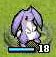# 星之一角

What have you found for these years?

## 2014-12-27

Just a copy from: ThreadGroup local variables

Here's the story. I wrote a testing framework which could run test
cases in parallel. To accumulate the number of assertions, I could
just use a shared number and lock it for each testing threads.

However, I would also like to detect if a single test case didn't
make any assertions, and raise an error. That means I can't just
lock the number, otherwise the thread won't have any idea if the
number was touched by the other threads. That means I need to lock
around each test cases, which defeats the purpose of running test
cases in parallel.

Then we could try to store the number inside the instance of the
running worker, something like this:

``````    def expect obj
Expect.new(obj) do
@assertions += 1
end
end

would 'test 1 == 1' do
@assertions = 0
expect(1).eq 1
end
``````
This works fine, but what if we want to make the other matcher,
such as `Kernel#should`, which has no idea about the worker?
``````    would 'test 1 == 1' do
@assertions = 0
1.should.eq 1
end
``````
Here 1 has absolutely no idea about the worker, how could it increment
the number then? We could try to use a thread local to accumulate the
assertions, and after all threads are done, accumulate all the numbers
from each threads. This way each numbers won't be interfering with each
number from the running thread local.

However this has an issue. What if a test case would spawn several
the worker thread local variable! Shown as below:
``````    would 'test 1 == 1' do
@assertions = 0
1.should.eq 1
end.join
end
``````
ThreadGroup to the rescue. Since a newly spawn thread would share the
same group from the parent thread, we could create a thread group for
each worker thread, and all objects should just find the corresponding
number by checking the thread group local. It should be protected by
a mutex, of course. Here's a demonstration:
``````    module Kernel
def should
Should.new(self) do
group[:assertions] += 1
end
# P.S. in the real code, it's a thread-safe Stat object
end
end
end
``````
Some alternative solutions:

* Just use instance_variable_set and instance_variable_get on ThreadGroup
* What I was doing before: Assume ThreadGroup#list.first is the owner of
the group, thus the worker thread, and use that thread to store the number.
Something like:
``Thread.current.group.list.first[:assertions] += 1``
This works for Ruby 1.9, 2.0, 2.1, but not for 2.2.
This also works for Rubinius. I thought this is somehow an expected behaviour,
therefore did a patch for JRuby to make this work:
https://github.com/jruby/jruby/pull/2221
Until now it failed on Ruby 2.2, did I know the order was not preserved...

* What I am doing right now: Find the worker thread through the list from the
group by checking the existence of the data from thread locals. Like:
``Thread.current.group.list.find{ |t| t[:assertions] }[:assertions] += 1``
At any rate, if we ever have thread group locals, the order won't be an issue,
at least for this use case.

Any idea?

#### 0 retries: